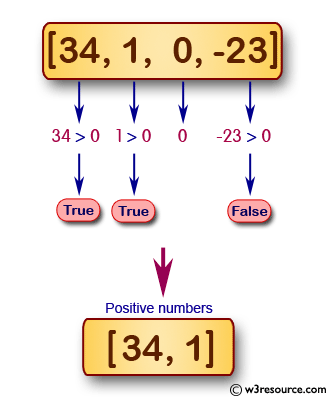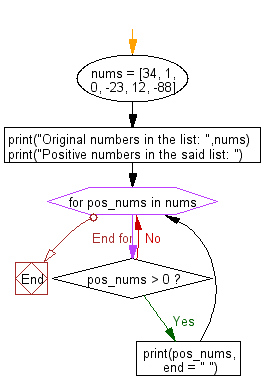﻿ Python: Filter the positive numbers from a list - w3resource# Python: Filter the positive numbers from a list

## Python Basic: Exercise-114 with Solution

Write a Python program to filter the positive numbers from a list.

Positive Numbers: Any number above zero is known as a positive number. Positive numbers are written without any sign or a '+' sign in front of them and they are counted up from zero, i.e. 1, + 2, 3, +4 etc.

Pictorial Presentation:Sample Solution-1:

Python Code:

``````nums = [34, 1, 0, -23, 12, -88]
print("Original numbers in the list: ",nums)
new_nums = list(filter(lambda x: x >0, nums))
print("Positive numbers in the said list: ",new_nums)
```
```

Sample Output:

```Original numbers in the list:  [34, 1, 0, -23, 12, -88]
Positive numbers in the said list:  [34, 1, 12]
```

## Visualize Python code execution:

The following tool visualize what the computer is doing step-by-step as it executes the said program:

Sample Solution-2:

Python Code:

``````nums = [34, 1, 0, -23, 12, -88]
print("Original numbers in the list: ",nums)
print("Positive numbers in the said list: ")
for pos_nums in nums:
if pos_nums > 0:
print(pos_nums, end = " ")
```
```

Sample Output:

```Original numbers in the list:  [34, 1, 0, -23, 12, -88]
Positive numbers in the said list:
34 1 12
```

Flowchart:## Visualize Python code execution:

The following tool visualize what the computer is doing step-by-step as it executes the said program:

Sample Solution-3:

Python Code:

``````nums = [34, 1, 0, -23, 12, -88]
print("Original numbers in the list: ",nums)
pos_nums = [n for n in nums if n> 0]
print("Positive numbers in the said list: ",*pos_nums)
```
```

Sample Output:

```Original numbers in the list:  [34, 1, 0, -23, 12, -88]
Positive numbers in the said list:  34 1 12
```

## Visualize Python code execution:

The following tool visualize what the computer is doing step-by-step as it executes the said program:

Python Code Editor:

Have another way to solve this solution? Contribute your code (and comments) through Disqus.

What is the difficulty level of this exercise?

Test your Python skills with w3resource's quiz

﻿

## Python: Tips of the Day

Try-catch-else construct:

```try:
foo()
except Exception:
print("Exception occured")
else:
print("Exception didnt occur")
finally:
print("Always gets here")
```